International
Tables for
Crystallography
Volume B
Reciprocal space
Edited by U. Shmueli

International Tables for Crystallography (2006). Vol. B. ch. 4.5, p. 469   | 1 | 2 |

## Section 4.5.2.4.1. Noncrystalline fibres

R. P. Millanea*

#### 4.5.2.4.1. Noncrystalline fibres

| top | pdf |

A noncrystalline fibre is made up of a collection of helical molecules that are oriented parallel to each other, but are otherwise randomly positioned and rotated relative to each other. The recorded intensity,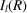, is therefore that diffracted by a single molecule cylindrically averaged about the Z axis in reciprocal space i.e.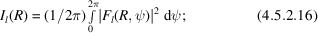using equation (4.5.2.12)shows that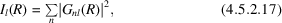where, as usual, the sum is over the values of n that satisfy the helix selection rule. On the diffraction pattern, reciprocal space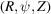collapses to the two dimensions (R, Z). The R axis is called the equator and the Z axis the meridian. The layer planes collapse to layer lines, at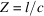, which are indexed by l. Equation (4.5.2.17)gives a rather simple relationship between the recorded intensity and the Fourier–Bessel structure factors.

Coherence length and disorientation, as described in Section 4.5.2.2, also affect the form of the diffraction pattern. These effects are described here, although they also apply to other than noncrystalline fibres. A finite coherence length leads to smearing of the layer lines along the Z direction. If the average coherence length of the molecules is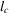, the intensity distribution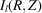about the lth layer line can be approximated by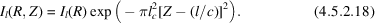It is convenient to express the effects of disorientation on the intensity distribution of a fibre diffraction pattern by writing the latter as a function of the polar coordinates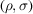(where σ is the angle with the Z axis) in (R, Z) space. Assuming a Gaussian orientation density function [equation (4.5.2.1)], ifis small and the effects of disorientation dominate over those of coherence length (which is usually the case except close to the meridian), then the distribution of intensity about one layer line can be approximated by (Holmes & Barrington Leigh, 1974; Stubbs, 1974)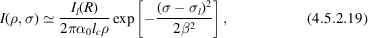where (Millane & Arnott, 1986; Millane, 1989c)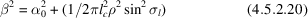and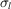is the polar angle at the centre of the layer line, i.e.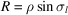. The effect of disorientation, therefore, is to smear each layer line about the origin of reciprocal space.

### ReferencesHolmes, K. C. & Barrington Leigh, J. (1974). The effect of disorientation on the intensity distribution of non-crystalline fibres. I. Theory. Acta Cryst. A30, 635–638.Google ScholarMillane, R. P. (1989c). Relating reflection boundaries in X-ray fibre diffraction patterns to specimen morphology and their use for intensity measurement. J. Macromol. Sci. Phys. B28, 149–166.Google ScholarMillane, R. P. & Arnott, S. (1986). Digital processing of X-ray diffraction patterns from oriented fibres. J. Macromol. Sci. Phys. B24, 193–227.Google ScholarStubbs, G. J. (1974). The effect of disorientation on the intensity distribution of non-crystalline fibres. II. Applications. Acta Cryst. A30, 639–645.Google Scholar